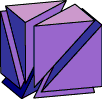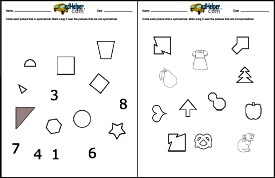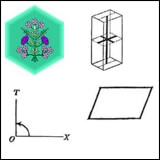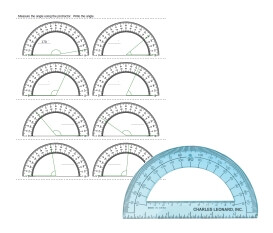Worksheets and No Prep Teaching Resources Math Worksheets Geometry is a great subject for students from elementary through middle school, and these worksheets will inspire them through innovative approaches to symmetry, transformations, plane figures, and more. These dynamic worksheets also cover using a protractor to learning fundamentals of rays and angles with the added bonuses of fun approaches to classifying plane figures, all aspects of lines and line segments, and exploring the exciting frontiers of perimeter, area, volume, and graphing. Get ready for high school geometry with these challenging, yet fun, worksheets that can be tailored to every student!Elementary to Middle School Geometry
Symmetry and Lines of SymmetrySymmetry and Lines of Symmetry

Transformations: Slides, Turns, Flips, and More

BooksThe ABCs of Geometry (Grades 4-7)

Plane Figures
Name each figure using letters and symbols
Draw and label an example of each
Classify each pair of lines as parallel, intersecting, or perpendicular
Classify each angle as acute, obtuse, straight, or right
Classify polygons
Classify triangles
Classify triangles according to lengths
Circles
Plane Figures Mixed Review

Drawing Shapes Challenge Lesson - Dot Game

Congruent Shapes and Figures

Lines and Line Segments

Measure Length of Line Segments

Using a Protractor and AnglesAngle activities using a protractor

Rays and Angles

Angles

Lines and Angles
Naming angle pairs (easy)
Naming angle pairs (pictures with 4 angles)
Angle pairs: Find the unknown angle measure
Naming adjacent, complementary, supplementary, and vertical angles
Find the unknown angle measure (pictures with 5 angles, one center)
Naming alternate interior/exterior angles, vertical angles, and corresponding angles
Find the unknown angle measure (traversals with parallel lines)
Lines and Angles Mixed Review

Perimeter

Area

Area and Perimeter (Grades 4-5)
Find the perimeter of each figure
Find the perimeter of each figure using a ruler
Perimeter: Find the value of n
Find the area of each figure
Find the value of n
Find the area and perimeter of each rectangle (no graphics)
Find the perimeter of a complex polygon (composed of 2 rectangles)
Find the perimeter of a complex polygon (composed of 3 rectangles)
Find the perimeter of a complex polygon (composed of 4 rectangles)
Find the area and perimeter of a complex polygon (composed of 2 rectangles)
Find the area and perimeter of a complex polygon (composed of 3 rectangles)
Find the area and perimeter of a complex polygon (composed of 4 rectangles)
Area and Perimeter Mixed Review (Grade 4)

Part 2
Area of a parallelogram (lengths are whole numbers)
Area of a parallelogram (lengths are decimals)
Area of a parallelogram (lengths are fractions)
Area of a triangle (lengths are whole numbers)
Area of a triangle (lengths are decimals)
Area of a triangle (lengths are fractions)
Find the circumference (graphics; lengths are whole numbers)
Find the circumference (graphics; lengths are decimals)
Find the circumference (graphics; lengths are fractions)
Find the circumference (lengths are whole numbers)
Find the circumference (lengths are decimals)
Find the circumference (lengths are fractions)
Area and Perimeter Mixed Review (Grade 5)

Area and Circumference of a Circle (Grades 6-7)
Perfect Squares and Square Roots
Write each perfect square, using the power 2
Write each perfect square as a square root
Find the square root
Find the value of n
Estimate a square root (multiple choice)
Circumference
Find the circumference (graphics; lengths are whole numbers)
Find the circumference (graphics; lengths are decimals)
Find the circumference (graphics; lengths are fractions)
Find the circumference (lengths are whole numbers)
Find the circumference (lengths are decimals)
Find the circumference (lengths are fractions)
Area of a Circle
Area of a circle (graphics; lengths are whole numbers)
Area of a circle (graphics; lengths are decimals)
Area of a circle (graphics; lengths are fractions)
Area of a circle (lengths are whole numbers)
Area of a circle (lengths are decimals)
Area of a circle (lengths are fractions)
Find the area of the partial circles
Review
Area and Circumference of a Circle Mixed Review

Coordinate Graphing
Coordinate Plane and Functions
Write the coordinates for each point
Finding ordered pairs
Drawing ordered pairs
Complete the function table
Complete the function table and then graph the function
Make a function from a line
Mixed Review
Transformations
Translations
Reflections
Rotations
Mix of translations, reflections, and rotations
Do multiple translations at once
Review
Coordinate Graphing Mixed Review

Test Prep
Grade 3 Geometry Test Prep
Grade 4 Geometry Test Prep
Grade 5 Geometry Test Prep
Grade 6 Geometry Test Prep
Grade 7 Geometry Test Prep

Perimeter, Circumference, and Area (middle school)
Find area of triangles (given coordinates)
Find area of rectangles and squares (given coordinates)
Find area of trapezoids (given coordinates)
Find area review (given coordinates)
Area and perimeter word problems: rectangles and parallelograms
Area and perimeter: rectangles with fractions
Area and perimeter of rectangles (algebra)
Area and perimeter: triangles
Area and perimeter: trapezoids
Area and perimeter: triangles (with fractions)
Area and perimeter: trapezoids (with fractions)
Pythagorean theorem
Circles: Find the circumference
Circles: Find the area
Circles: Find the circumference and area
Circles: Find the radius (algebra)
Perimeter, Circumference, and Area Review Printable

Volume and Surface Area
Surface area and volume of right rectangular prisms
Volume of prisms and cylinders (Graphics)
Volume of cones, pyramids, and spheres (Graphics)
Volume of prisms, cylinders, cones, pyramids, and spheres
Surface area of prisms and cylinders
Surface area of pyramids, cones, and spheres
Volume and Surface Area Review Printable

Have a suggestion or would like to leave feedback?